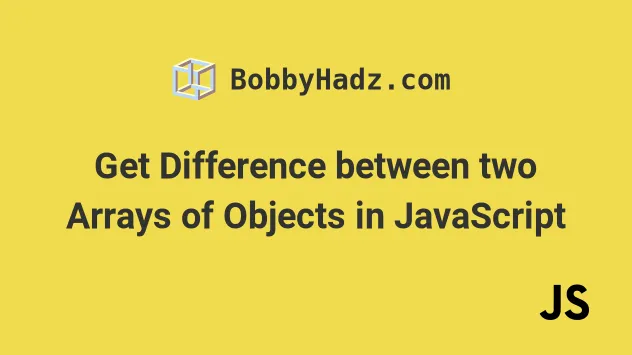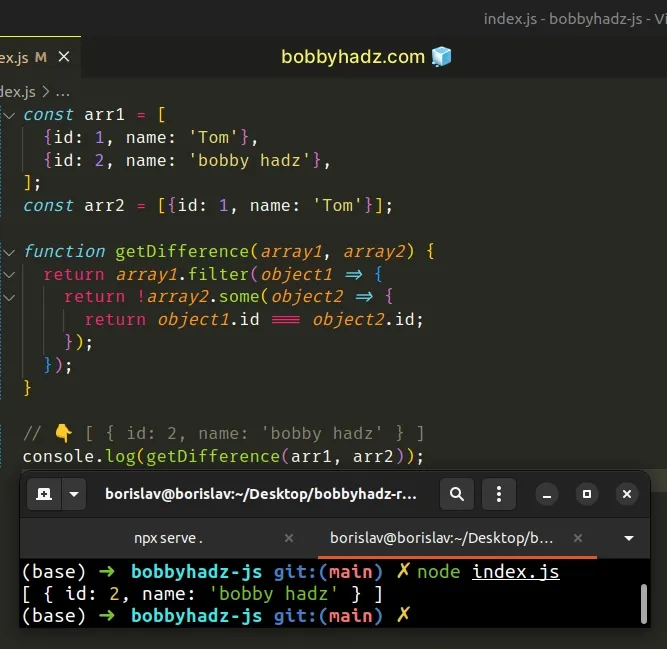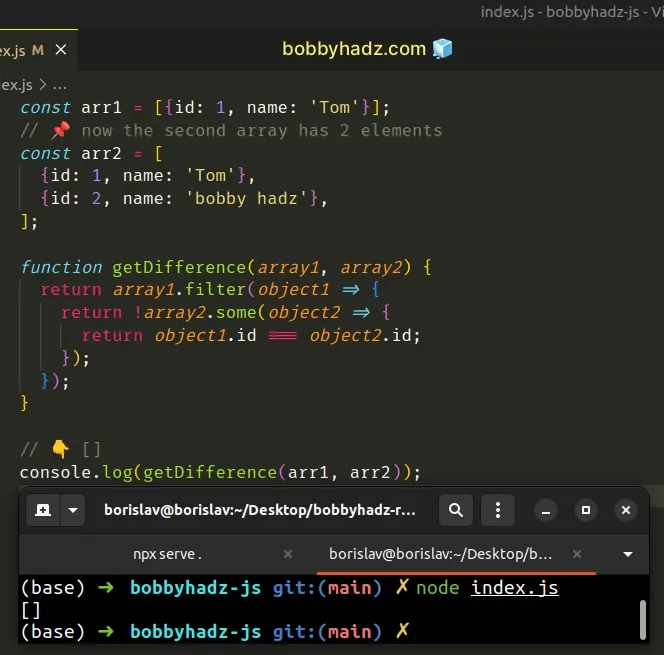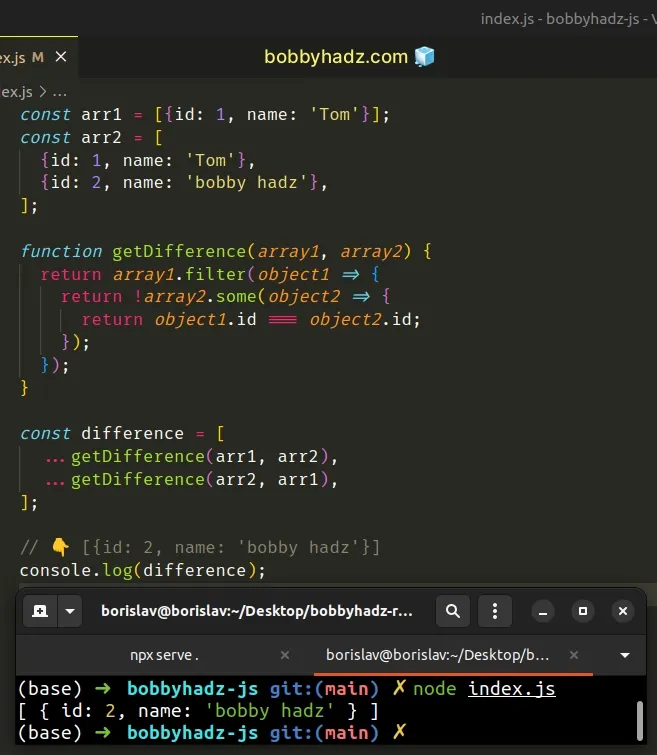# Get Difference between two Arrays of Objects in JavaScriptLast updated: Jan 10, 2023
3 min## #Get the Difference between two Arrays of Objects in JS

To get the difference between two arrays of objects:

1. Use the `filter()` method to iterate over the first array.
2. Check if each object is not contained in the second array.
3. Repeat steps 1 and 2 for the second array.
4. Concatenate the results to get the complete difference.
index.js
```Copied!```const arr1 = [
{id: 1, name: 'Tom'},
];
const arr2 = [{id: 1, name: 'Tom'}];

function getDifference(array1, array2) {
return array1.filter(object1 => {
return !array2.some(object2 => {
return object1.id === object2.id;
});
});
}

// 👇️ [ { id: 2, name: 'bobby hadz' } ]
console.log(getDifference(arr1, arr2));
``````The function we passed to the Array.filter method gets called with each element (object) in the array.

On each iteration, we use the Array.some() method on the second array.

The function we passed to the `some()` method also gets invoked with each element (object) in the array.

The `some()` method tests whether at least one element in the array passes a condition.

We check if at least `1` object from the first array has the same `id` property as an object from the second array.

We used the logical NOT (!) operator to negate the result from the `some()` method because we only care about objects that don't have the same `id` property between the arrays.

If the condition is met, the `some()` method returns `true` and the object gets included in the array that the `filter()` method returns.

## #Get the complete difference between two Arrays of Objects

However, we only called the `filter` method on one of the arrays. So what happens if the first array contains fewer elements than the second?

index.js
```Copied!```const arr1 = [{id: 1, name: 'Tom'}];
// 📌 now the second array has 2 elements
const arr2 = [
{id: 1, name: 'Tom'},
];

function getDifference(array1, array2) {
return array1.filter(object1 => {
return !array2.some(object2 => {
return object1.id === object2.id;
});
});
}

// 👇️ []
console.log(getDifference(arr1, arr2));
``````The only thing we changed is we switched the values of the `arr1` and `arr2` variables.

Now we call the `filter()` method on the first array.

However, the only object contained in the array is also contained in the second array, so the `getDifference()` function returns an empty array.

We would expect the return value to be `[{id: 2, name: 'bobby hadz'}]`.

We need to call the `getDifference()` function two times and combine the results to get the complete difference.

index.js
```Copied!```const arr1 = [{id: 1, name: 'Tom'}];
const arr2 = [
{id: 1, name: 'Tom'},
];

function getDifference(array1, array2) {
return array1.filter(object1 => {
return !array2.some(object2 => {
return object1.id === object2.id;
});
});
}

const difference = [
...getDifference(arr1, arr2),
...getDifference(arr2, arr1)
];

// 👇️ [{id: 2, name: 'bobby hadz'}]
console.log(difference);
``````To get the complete difference between two arrays of objects:

1. Call the `filter` method on the first array and return only the objects that are not contained in the second array.
2. Call the `filter` method on the second array and return only the objects that are not contained in the first array.
3. Combine the two arrays into a third array using the spread syntax (...).

An easy way to think about the spread syntax (...) is that we are unpacking the values of one array into another array.

Now our example returns the complete difference between the two arrays of objects.

You can also shorten the `getDifference` function by using arrow functions with implicit returns.

index.js
```Copied!```const arr1 = [{id: 1, name: 'Tom'}];
const arr2 = [
{id: 1, name: 'Tom'},
];

function getDifference(array1, array2) {
return array1.filter(
object1 => !array2.some(
object2 => object1.id === object2.id
),
);
}

const difference = [
...getDifference(arr1, arr2),
...getDifference(arr2, arr1)
];

// 👇️ [{id: 2, name: 'bobby hadz'}]
console.log(difference);
``````

We removed the curly braces and explicit `return` statements from the `getDifference` function to make it a little more concise.

I've also written an article on how to get the difference between two arrays in JS.